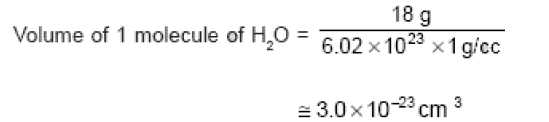Question 1

The work function of a surface of a photosensitive material is 6.2 eV. The wavelength of the incident radiation for which the stopping potential is 5 V lies in the

A : X-ray region
B : Ultraviolet region
C : Visible region
D : Infrared region

•
.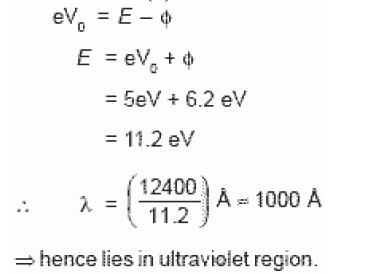•   Question 2

A particle of mass 1 mg has the same wavelength as an electron moving with a velocity of 3 � 106 ms-1. The velocity of the particle is (mass of electron = 9.1 * 10-31 kg)

A : 2.7 * 10-21 ms-1
B : 2.7 * 10-18 ms-1
C : 9 * 10-2 ms-1
D : 3 * 10-31 ms-1

•
.

Solution :
same momentum of both the particles

•   Question 3

The ground state energy of hydrogen atom is -13.6 eV. When its electron is in the first excited state, its excitation energy is

A : 0
B : 3.4eV
C : 6.8eV
D : 10.2eV

•
.

Solution :
Excitation energy = Ef - Ei = -3.4 - (-13.6) = 10.2 eV

•   Question 4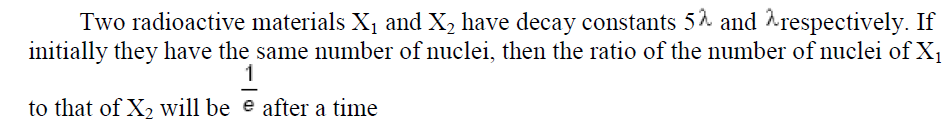A :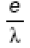B :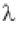C :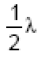D :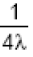•
.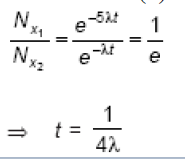•   Question 5

Two nuclei have their mass numbers in the ratio of 1:3. The ratio of their nuclear densities would be

A : 1 : 1
B : 1 : 3
C : 3 : 1
D : (3)^1/3 : 1

•
.

Solution :
Density is independent of mass number of nuclei.

•   Question 6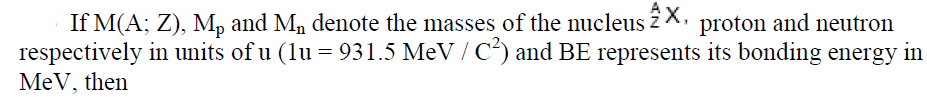A :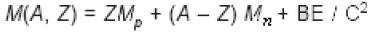B :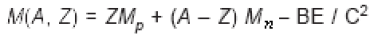C :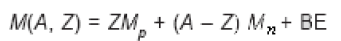D :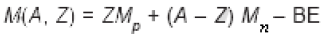•
.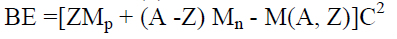•   Question 7

The voltage gain of an amplifier with 9% negative feedback is 10. The voltage gain without feedback will be

A : 100
B : 90
C : 10
D : 1.25

•
.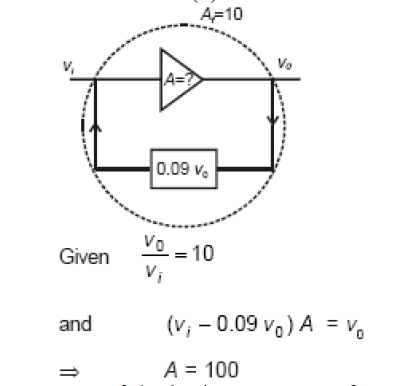•   Question 8

If the lattice parameter for a crystalline structure is 3.6 A , then the atomic radius in fcc crystal is

A : 1.27A
B : 1.81A
C : 2.10A
D : 2.92A

•
.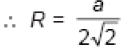•   Question 9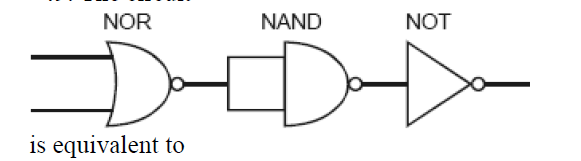A : OR GATE
B : AND GATE
C : NAND GATE
D : NOR GATE

•
.

Solution :
simplifies to NOR GATE

•   Question 10

A p-n photodiode is made of a material with a band gap of 2.0 eV. The minimum frequency of the radiation that can be absorbed by the material is nearly

A : 20 * 1014 Hz
B : 10 * 1014 Hz
C : 5 * 1014 Hz
D : 1 * 1014 Hz

•
.

Solution :
v=E/H = 5 * 10 14 Hz

•   Question 11

If uncertainty in position and momentum are equal, then uncertainty in velocity is

A :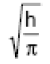B :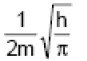C :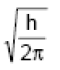D :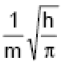•
.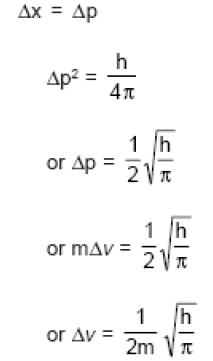•   Question 12

If a gas expands at constant temperature, it indicates that

A : Number of the molecules of gas increases
B : Kinetic energy of molecules decreases
C : Pressure of the gas increases
D : Kinetic energy of molecules remains the same

•
.

Solution :
Kinetic energy of gaseous molecules depends on temperature only

•   Question 13

The value of equilibrium constant of the reaction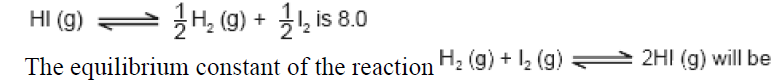A : 1/8
B : 1/16
C : 1/64
D : 16

•
.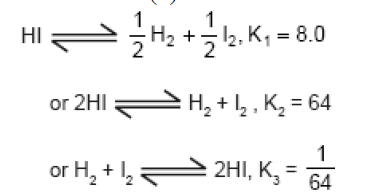•   Question 14

If 'a' stands for the edge length of the cubic systems : simple cubic, body centred cubic and face centred cubic, then the ratio of radii of the spheres in these systems will be respectively

A :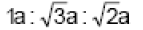B :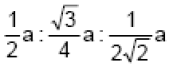C :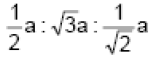D :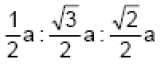•
.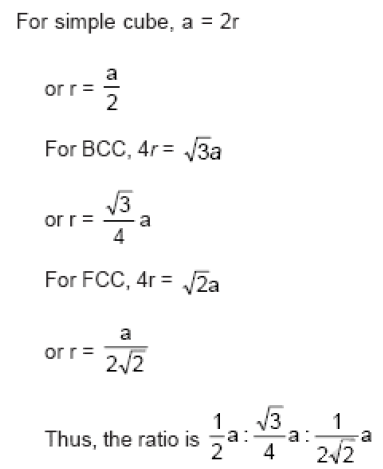•   Question 15

Kohlrausch's law states that at

A : Infinite dilution, each ion makes definite contribution to equivalent conductance of an electrolyte, whatever be the nature of the other ion of the electrolyte
B : Finite dilution, each ion makes definite contribution to equivalent conductance of an electrolyte, whatever be the nature of the other ion of the electrolyte
C : Infinite dilution each ion makes definite contribution to equivalent conductance of an electrolyte depending on the nature of the other ion of the electrolyte
D : Infinite dilution, each ion makes definite contribution to conductance of an electrolyte whatever be the nature of the other ion of the electrolyte

•
.

Solution :
Kohlrausch's law states, ''at infinite dilution each ion contributes its fixed value towards equivalent conductance irrespective of the other ion in combination with it at fixed temperature.''

•   Question 16

The measurement of the electron position is associated with an uncertainty in momentum, which is equal to 1 * 10-18 g cm s-1. The uncertainty in electron velocity is, (mass of an electron is 9 � 10^-28 g)

A : 1 * 1011 cm s-1
B : 1 * 109 cm s-1
C : 1 * 106 cm s-1
D : 1 * 105 cm s-1

•
.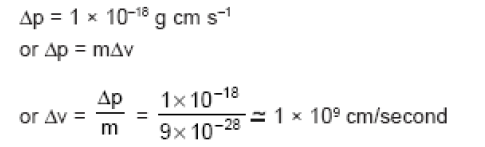•   Question 17

Which of the following are not state functions?
(I) q + w
(II) q
(III) w
(IV) H-TS

A : (II) and (III)
B : (I) and (IV)
C : (II), (III) and (IV)
D : (I), (II) and (III)

•
.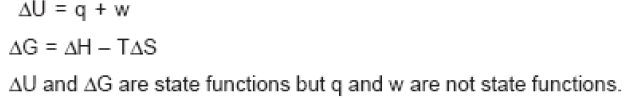•   Question 18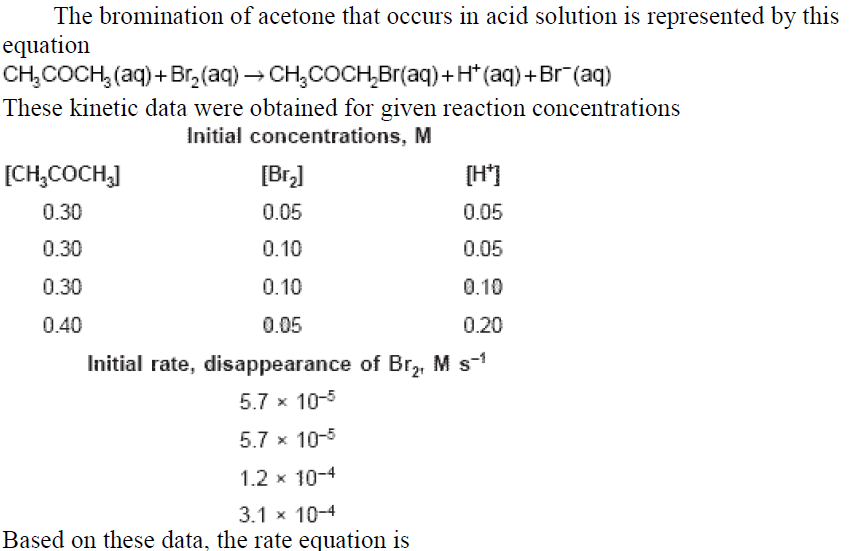A :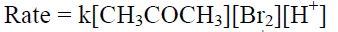B :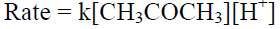C :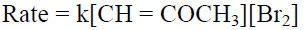D :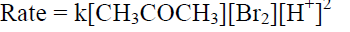•
.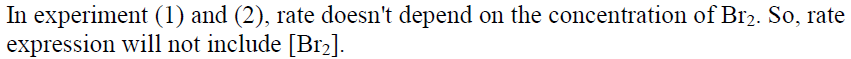•   Question 19

What volume of oxygen gas (O2) measured at 0 deg C and 1 atm, is needed to burn completely 1 L of propane gas (C3H8) measured under the same conditions?

A : 10L
B : 7L
C : 6L
D : 5L

•
.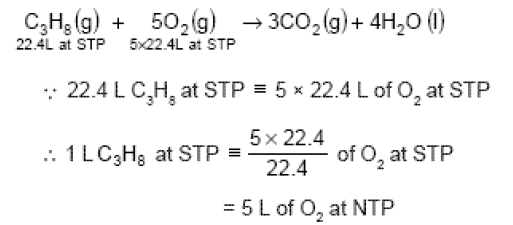•   Question 20

Bond dissociation enthalpy of H2, Cl2 and HCl are 434, 242 and 431 kJmol- 1respectively. Enthalpy of formation of HCl is

A : 245 kJmol-1
B : 93 kJmol-1
C : -245 kJmol-1
D : -93 kJmol-1

•
.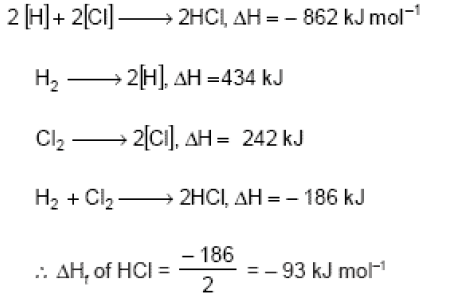•   Question 21

Which of the following statements is not correct?

A : The number of Bravais lattices in which a crystal can be categorized is 14
B : The fraction of the total volume occupied by the atoms in a primitive cell is 0.48
C : Molecular solids are generally volatile
D : The number of carbon atoms in an unit cell of diamond is 4

•
.

Solution :
Diamond has ZnS type structure. So, no. of atoms in a unit cell of diamond is 8. Note : But option (2) is also incorrect because the fraction of the total volume occupied by the atoms in a cubic primitive cells is 0.524

•   Question 22

Equal volumes of three acid solutions of pH 3, 4 and 5 are mixed in a vessel. What will be the H+ ion concentration in the mixture?

A : 1.11 * 10-3 M
B : 1.11 * 10-4 M
C : 3.7 * 10-4 M
D : 3.7 * 10-3 M

•
.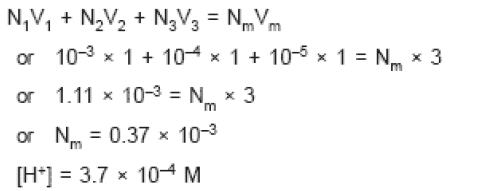•   Question 23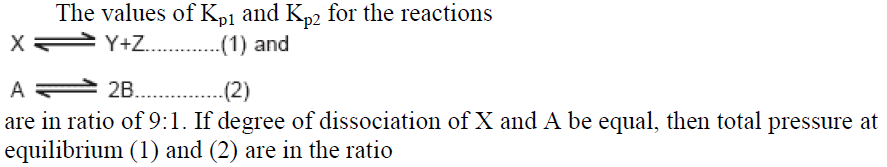A : 1 : 1
B : 3 : 1
C : 1 : 9
D : 36 : 1

•
.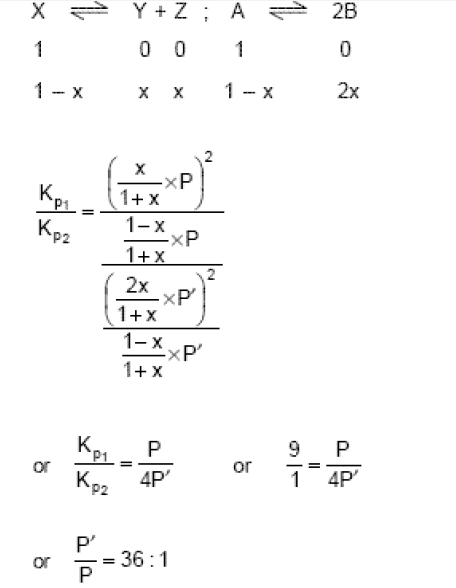•   Question 24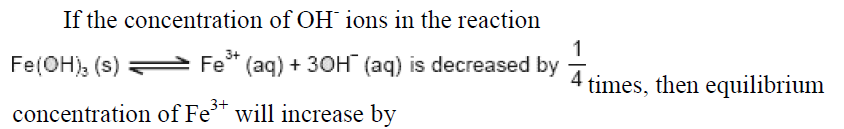A : 4 times
B : 8 times
C : 16 times
D : 64 times

•
.

Solution :
To maintain the constant value of Kc, the concentration of Fe3+ ion will increase by 64 times.

•   Question 25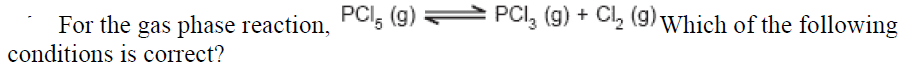A :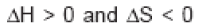B :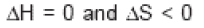C :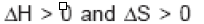D :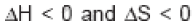•
.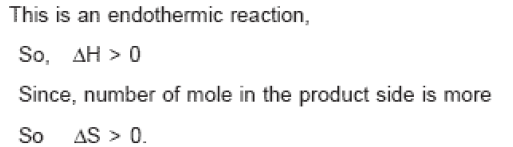•   Question 26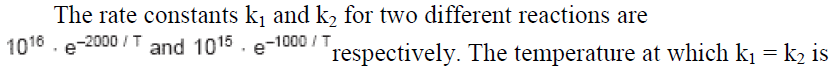A :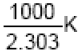B :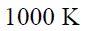C :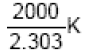D :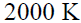•
.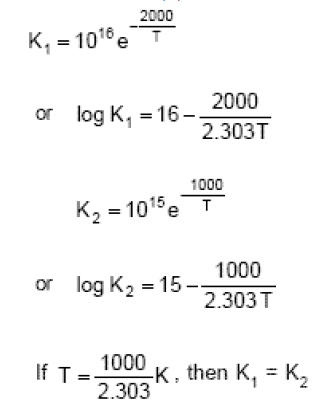•   Question 27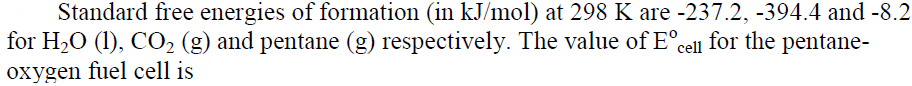A : 0.0968 V
B : 1.968 V
C : 2.0968 V
D : 1.0968 V

•
.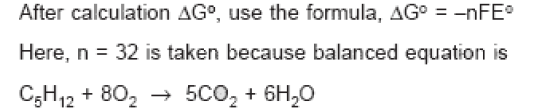•   Question 28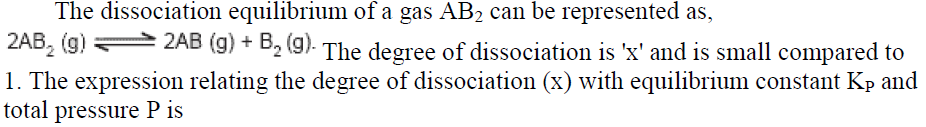•
.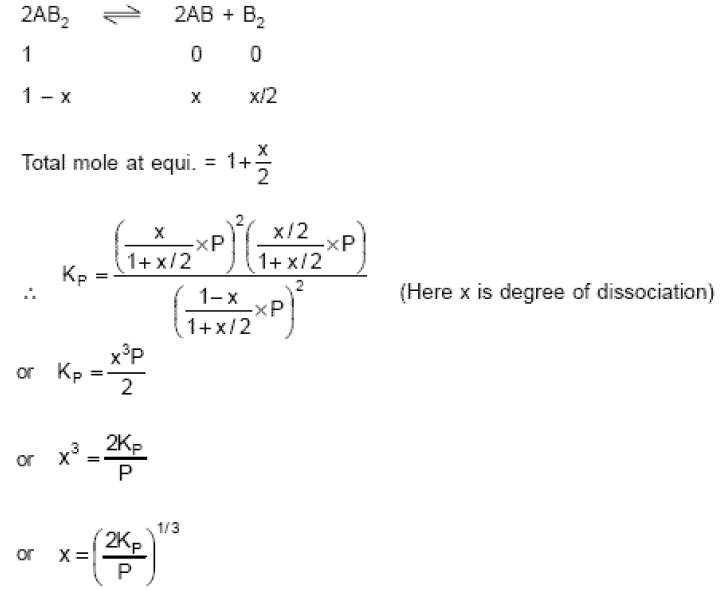•   Question 29

The sequence of ionic mobility in aqueous solution is

A : Na+ > K+ > Rb+ > Cs+
B : K+ > Na+ > Rb+ > Cs+
C : Cs+ > Rb+ > K+ > Na+
D : Rb+ > K+ > Cs+ > Na+

•
.

Solution :
More the charge density of ion, more will be the ion-dipole interaction, so more will be hydration of ion and hence less will be the ionic mobility.

•   Question 30

Percentage of free space in a body centred cubic unit cell is

A : 28%
B : 30%
C : 32%
D : 34%

•
.

•   Question 31

The correct order of decreasing second ionisation enthalpy of Ti (22), V (23), Cr (24) and Mn (25) is

A : Ti > V > Cr > Mn
B : Cr > Mn > V > Ti
C : V > Mn > Cr > Ti
D : Mn > Cr > Ti > V

•
.

Solution :
After removing one electron from chromium, the resulting structure becomes more stable. Hence Cr has higher second ionisation enthalpy. Thus, the correct order is Cr > Mn > V > Ti

•   Question 32

How many moles of lead (II) chloride will be formed from a reaction between 6.5 g of PbO and 3.2 g of HCl?

A : 0.029
B : 0.044
C : 0.333
D : 0.011

•
.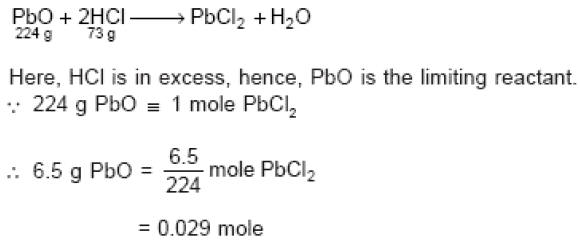•   Question 33

Which of the following complexes exhibits the highest paramagnetic behaviour?
Where gly = glycine, en = ethylenediamine and bpy = bipyridyl moities). (At. number Ti = 22, V = 23, Fe = 26, Co = 27)

A :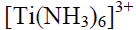B :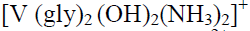C :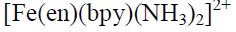D :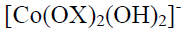•
.•   Question 34

Volume occupied by one molecule of water (density = 1 g cm-3) is

A : 5.5 * 10-23 cm3
B : 9.0 * 10-23 cm3
C : 6.023 * 10-23 cm3
D : 3.0 * 10-23 cm3

•
.